﻿ 传热学课程中黑体辐射五大定律关系阐述分析

# 传热学课程中黑体辐射五大定律关系阐述分析Describing and Analysis of the Relationship among Five Laws of Blackbody Radiation in the Course of “Heat Transfer”

Abstract: Thermal radiation is one of the most basic and complex heat transfer modes in “Heat Transfer”. This paper will demonstrate the exploration and practice in the teaching practice using the five radiative laws of the blackbody radiation as an example. From two aspects of graphic demonstration and math formulation derivation, the relationship of the five laws will be described and analyzed in order to deepen students' understanding of the radiative heat transfer, and try to arouse their interest in learning and research.

1. 引言

“传热学”是研究由温差引起的热能传递规律的科学，热辐射是“传热学”课程中最基本、最复杂的一种传热方式  。只要当物体的温度大于0 K，物体就会发出热辐射，因此它也是一种处处存在的传热方式。由于热辐射的复杂性，人们从19世纪才开始对这种传热方式进行研究，而在“传热学”授课过程中，学生对于热辐射的理解往往比较浅显，仅仅依靠课堂教学很难使学生正确理解基本规律和公式    。为此，笔者在授课过程中有意扩展这方面的讲解和实践，试图加强学生对热辐射的理解和认识。

2. 黑体辐射五大定律图形关系

${E}_{b\lambda }=\frac{{c}_{1}{\lambda }^{-5}}{{\text{e}}^{{c}_{2}/\left(\lambda T\right)}-1}$ (1)

2.1. 普朗克定律、维恩公式和瑞利–金斯公式间的图形关系

${E}_{b\lambda }\left(T\right)={C}_{1}{\lambda }^{-5}{\text{e}}^{-\frac{{C}_{2}}{\lambda T}}$ (2)

${E}_{b\lambda }\left(T\right)={C}_{3}{\lambda }^{-4}T$ (3)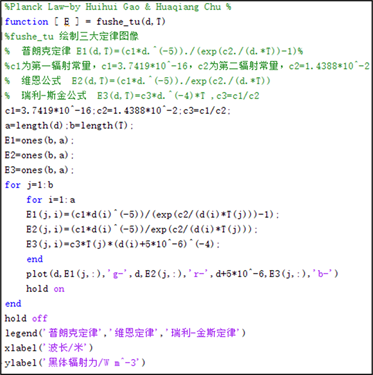(a)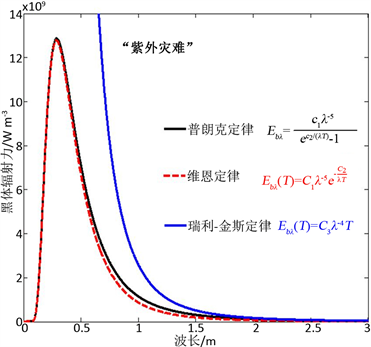(b)

Figure 1. Matlab code and the relationship between the three laws

2.2. 普朗克定律、维恩位移定律和斯忒藩-玻尔兹曼定律间的图形关系

${\lambda }_{m}T=2.8978×{10}^{-3}\text{\hspace{0.17em}}\text{m}\cdot K$ (4)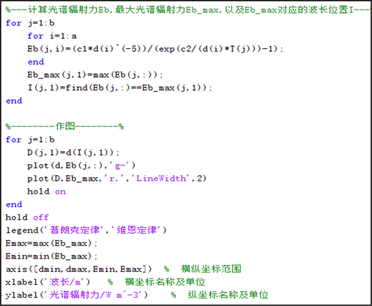(a)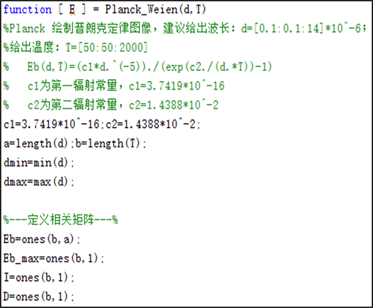(b)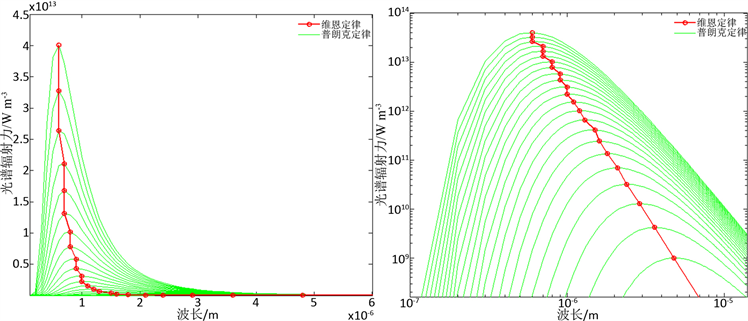(c) (d)

Figure 2. Matlab code and Graphic Relations between Planck’s Law and Venn’s law of displacement ((c), (d))

${E}_{b}=\sigma {T}^{4}$ (5)(a) (b)

Figure 3. Graphic Relations between Planck's Law and Stefan-Boltzmann’s Law

3. 黑体辐射五大定律公式推导

3.1. 普朗克定律、维恩公式和瑞利–金斯公式间的关系推导

${c}_{2}\ll \lambda T$ ，即当 ${\text{e}}^{{c}_{2}/\left(\lambda T\right)}\ll 1$ 时，对 ${\text{e}}^{{c}_{2}/\left(\lambda T\right)}$${c}_{2}/\lambda T=0$ 处进行泰勒展开有， ${\text{e}}^{{c}_{2}/\left(\lambda T\right)}={{\text{e}}^{{c}_{2}/\left(\lambda T\right)}|}_{{c}_{2}/\left(\lambda T\right)=0}+{{\left({\text{e}}^{{c}_{2}/\left(\lambda T\right)}\right)}^{\prime }|}_{{c}_{2}/\left(\lambda T\right)=0}\frac{{c}_{2}}{\lambda T}+\cdots =1+\frac{{c}_{2}}{\lambda T}+\cdots$ ，忽略二阶项后将此式代入普朗克定律即可得瑞利–金斯公式： ${E}_{b\lambda }=\frac{{c}_{1}{\lambda }^{-5}}{{\text{e}}^{{c}_{2}/\left(\lambda T\right)}-1}\approx \frac{{c}_{1}{\lambda }^{-5}}{1+\frac{{c}_{2}}{\lambda T}-1}={c}_{3}{\lambda }^{-4}T$ 。因此，在温度一定时，波长比较长情况下普朗克定律可近似为瑞利–金斯公式。

3.2. 普朗克定律、维恩位移定律和斯忒藩–玻尔兹曼定律间公式推导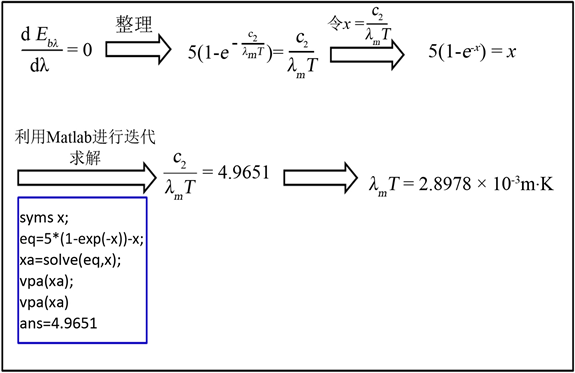Figure 4. Derived relations between Planck’s law and Wien’s law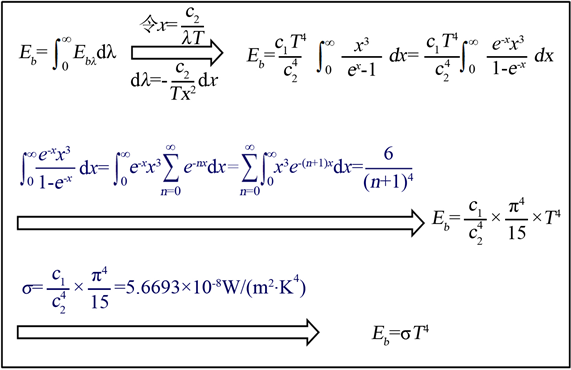Figure 5. Derived relations between Planck’s Law and Stefan-Boltzmann’s Law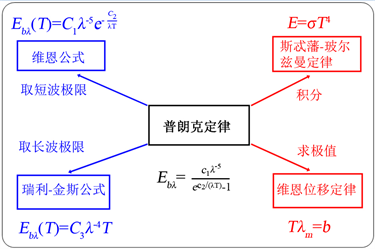Figure 6. Correlation between five laws of blackbody radiation

4. 结束语

 杨世铭, 陶文铨. 传热学[M]. 北京: 高等教育出版社, 2004.

 高芬, 安莹, 董威. 基于MATLAB的黑体辐射量计算[J]. 光学与光电技术, 2005, 3(5): 30-32.

 徐代升, 王元樟. 基于图形的黑体辐射三大基本定律关系阐述[J]. 物理与工程, 2012, 22(5): 8-11.

 陈晓明. 黑体辐射定律及实验教学相关问题探讨[J]. 实验室研究与探索, 2009, 28(5): 27-29.

 Stormy Attaway. MATLAB编程与工程应用[M]. 第二版. 北京: 电子工业出版社, 2013.

Top